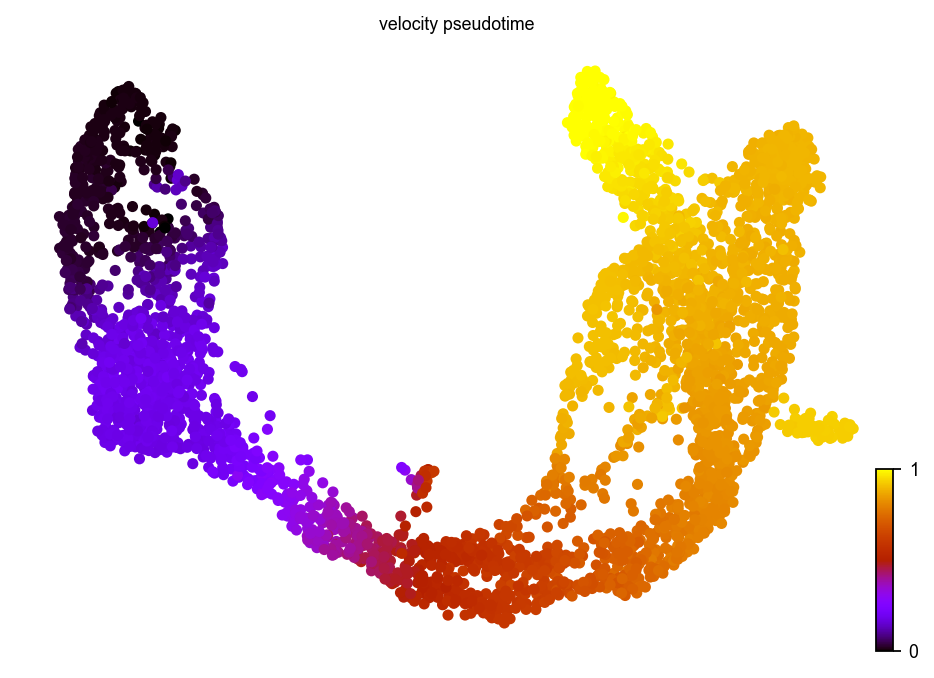# scvelo.tl.velocity_pseudotime¶

`scvelo.tl.``velocity_pseudotime`(adata, vkey='velocity', groupby=None, groups=None, root_key=None, end_key=None, n_dcs=10, use_velocity_graph=True, save_diffmap=None, return_model=None, **kwargs)

Computes a pseudotime based on the velocity graph.

Velocity pseudotime is a random-walk based distance measures on the velocity graph. After computing a distribution over root cells obtained from the velocity-inferred transition matrix, it measures the average number of steps it takes to reach a cell after start walking from one of the root cells. Contrarily to diffusion pseudotime, it implicitly infers the root cells and is based on the directed velocity graph instead of the similarity-based diffusion kernel.

```scv.tl.velocity_pseudotime(adata)
```adata: `AnnData`

Annotated data matrix

vkey: str (default: ‘velocity’)

Name of velocity estimates to be used.

groupby: str, list or np.ndarray (default: None)

Key of observations grouping to consider.

groups: str, list or np.ndarray (default: None)

Groups selected to find terminal states on. Must be an element of adata.obs[groupby]. Only to be set, if each group is assumed to have a distinct lineage with an independent root and end point.

root_key: int (default: None)

Index of root cell to be used. Computed from velocity-inferred transition matrix if not specified.

end_key: int (default: None)

Index of end point to be used. Computed from velocity-inferred transition matrix if not specified.

n_dcs: int (default: 10)

The number of diffusion components to use.

use_velocity_graph: bool (default: True)

Whether to use the velocity graph. If False, it uses the similarity-based diffusion kernel.

save_diffmap: bool (default: None)

Whether to store diffmap coordinates.

return_model: bool (default: None)

Whether to return the vpt object for further inspection.

**kwargs:

Further arguments to pass to VPT (e.g. min_group_size, allow_kendall_tau_shift).

Returns

velocity_pseudotime (.obs) – Velocity pseudotime obtained from velocity graph.